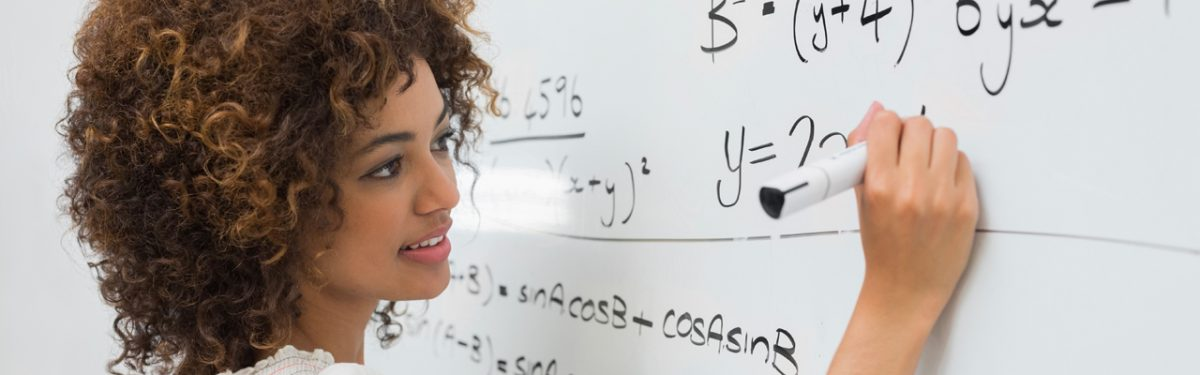# LINR 1 | Lesson 1 | Making Final Connections (Solutions)

## Making Final Connections

1. If you know a linear pattern is growing by 5, what other information would you need to determine the equation?    You need to know how many squares are in the zero step (or any step and count backwards) to determine the y-intercept.

2. If you know a linear pattern has 10 squares in step 4, what other information would you need to determine the equation?    You need to know the number of squares in another step.  Then, you can compute the slope and then count backwards to find the number of squares in the zero step.

3. If you know a pattern has 42 squares in step 1 and grows by 3, what is the equation?  The equation is $$s=3n+39$$ since there are 39 squares in the zero step $$(42-3=39)$$.